# Bitcoin Address Generator in Obfuscated Python

Recently, I became interested in the inner workings of Bitcoin – specifically, the way it uses elliptic curve cryptography to generate Bitcoin addresses such as 1PreshX6QrHmsWbSs8pHpz6kLRcj9kdPy6. It inspired me to write another obfuscated Python script. The following is valid Python code:

_                   =r"""A(W/2,*M(3*G
*G*V(2*J%P),G,J,G)+((M((J-T
)*V((G-S)%P),S,T,G)if(S@(G,J))if(
W%2@(S,T)))if(W@(S,T);H=2**256;import&h
ashlib&as&h,os,re,bi    nascii&as&k;J$:int( k.b2a_hex(W),16);C$:C    (W/    58)+[W%58]if(W@
[];X=h.new("rip           em    d160");Y$:h.sha25 6(W).digest();I$                 d=32:I(W/256,d-1)+
chr(W%256)if(d>0@"";                  U$:J(k.a2b_base 64(W));f=J(os.urando m(64)) %(H-U("AUVRIxl Qt1/EQC2hcy/JvsA="))+ 1;M$Q,R,G       :((W*W-Q-G)%P,
(W*(G+2*Q-W*W)-R)%P)       ;P=H-2**       32-977;V$Q=P,L= 1,O=0:V(Q%W,W,O-Q/W* L,L)if(W@O%P;S, T=A(f,U("eb5mfvncu6 xVoGKVzocLBwKb/Nst zijZWfKBWxb4F5g="), U("SDra dyajxGVdpPv8DhEI qP0XtEimhVQZnEfQj/ sQ1Lg="), 0,0);F$:"1"+F(W
[1:])if(W[:1           ]=="\0"@""        .join(map(B,C(
J(W))));K$: F(W +Y(Y(W))[:4]); X.update(Y("\4"+ I(S)+I(T)));B$
:re.sub("[0OIl    _]|            [^\\w]","","".jo
ss:",K("\0"+X.dig    est())+"\nPrivkey:",K(
"\x80"+I(f))""";exec(reduce(lambda W,X:
W.replace(*X),zip(" \n&$@",["","", " ","=lambda W,",")else "]) ,"A$G,J,S,T:"+_))


Python 2.5 – 2.7 is required. Each time you run this script, it generates a Bitcoin address with a matching private key.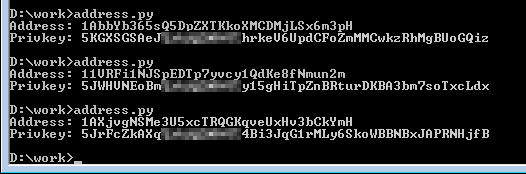So, what’s going on here? Basically, this little script gives you the ability to throw some money around. Obviously, I don’t recommend doing so. I just think it’s cool that such a thing is even possible. Allow me to demonstrate.

## Sending Bitcoins to One of These Addresses

To show that the above Python script generates working Bitcoin addresses, I’ll go ahead and send 0.2 BTC – that’s currently over \$100 worth – to the first address shown in the above screenshot. I’ll use Bitcoin-Qt, the original Bitcoin desktop wallet.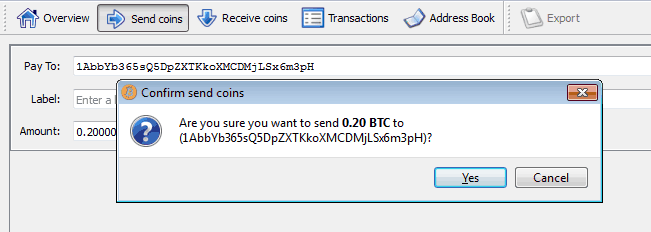Here’s the transaction verified on Blockchain.info. Goodbye, 0.2 BTC!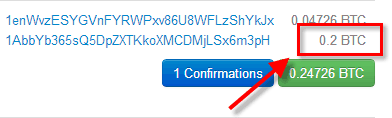Now, if I didn’t have the private key corresponding to 1AbbYb365sQ5DpZXTKkoXMCDMjLSx6m3pH, those bitcoins would be lost forever. Fortunately, I do have the private key. It was generated by the Python script too.

## Recovering Those Bitcoins

To recover those bitcoins, I’ll use another desktop wallet called Electrum. Under Wallet → Private keys → Import, I can enter the private key: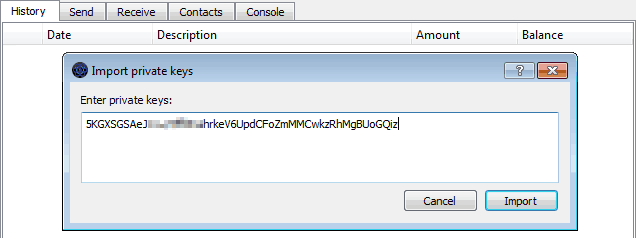…and presto! Electrum considers those 0.2 BTC mine to spend once again.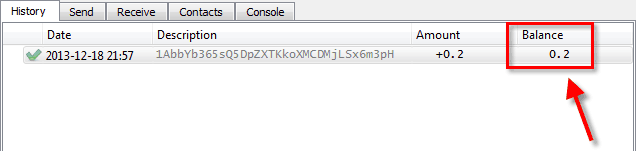To make sure, let’s send them back to another address.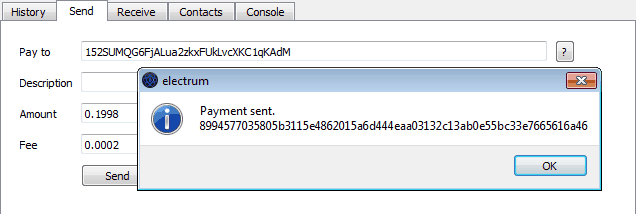Here’s the final transaction verified on Blockchain.info.

There you have it. We’ve successfully sent money to – and more importantly, back from – a Bitcoin address that was generated by some code shaped like a Bitcoin logo.

## What Does This Illustrate About Bitcoin?

Bitcoin addresses are created out of thin air. First, the script generates a pseudorandom number – that’s the private key. It then multiplies that number by an elliptic curve point to find the matching public key. The public key is shortened by a hash function, producing a Bitcoin address. Finally, both private key and address are encoded as text. Most Bitcoin wallet applications generate addresses in exactly this way.

Randomness ensures that each address is unique. With addresses created out of thin air, you might worry that two different Bitcoin wallets will eventually generate the same address. That’s not impossible, but with a strong pseudorandom number generator, it’s very, very, very, very, very, very, very, very unlikely. There are 2160 possible Bitcoin addresses. If you were to generate one million addresses per second for 5000 years, you’d be more likely to have a meteor fall on your house than to ever see the same address twice.